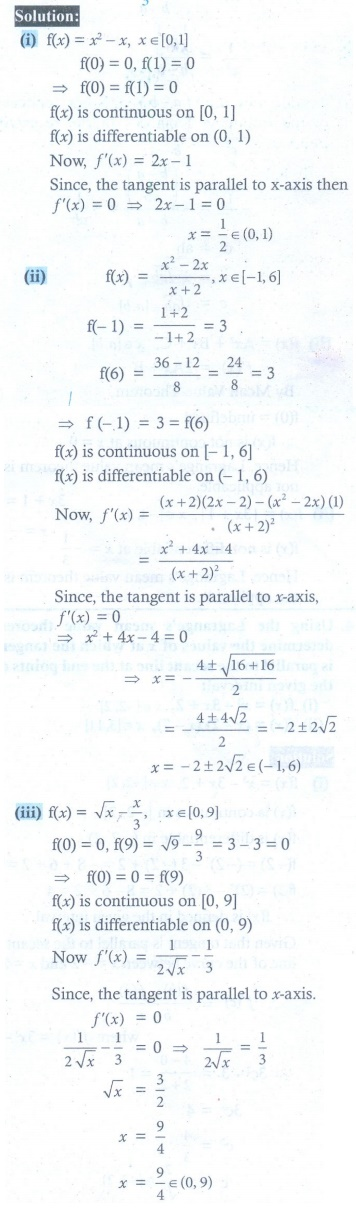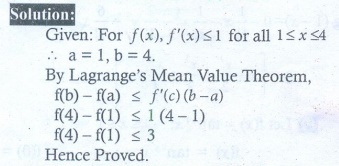Home | | Maths 12th Std | Exercise 7.3: Mean Value Theorem

# Exercise 7.3: Mean Value Theorem

Maths Book back answers and solution for Exercise questions - Mathematics : Applications of Differential Calculus : Mean Value Theorem

EXERCISE 7.3

1. Explain why RolleŌĆÖs theorem is not applicable to the following functions in the respective intervals.

(i) f (x) = |1/x| , x Ōłł[ŌłÆ1,1]

(ii) f (x) = tan x, x Ōłł[0,ŽĆ ]

(iii) (x) = x ŌłÆ 2 log x, x Ōłł[2, 7]2. Using the RolleŌĆÖs theorem, determine the values of x at which the tangent is parallel to the -axis for the following functions :

(i) (x) = x2 ŌłÆ x, x Ōłł[0,1]

(ii) f (x) = (x2 ŌłÆ 2x) / (x + 2), x Ōłł [ ŌłÆ1, 6]

(ii) f (x) = ŌłÜx ŌĆō x/3 , x Ōłł[0, 9]3. Explain why LagrangeŌĆÖs mean value theorem is not applicable to the following functions in the respective intervals :

(i) f (x) = x +1 / x, x Ōłł [ ŌłÆ1, 2]

(ii) f (x) = | 3x +1 |, x Ōłł [ ŌłÆ1, 3]4. Using the LagrangeŌĆÖs mean value theorem determine the values of x at which the tangent is parallel to the secant line at the end points of the given interval:

(i) f ( x= x3 ŌłÆ 3x + 2, x Ōłł[ŌłÆ2, 2]

(ii) f (x= ( x ŌłÆ 2)(x ŌłÆ 7), x Ōłł[3,11]5. Show that the value in the conclusion of the mean value theorem for

(i) f (x) = 1/x on a closed interval of positive numbers [a, b] is ŌłÜ(ab)

(ii) (x) = Ax2 + Bx + C on any interval [a, b] is (+b) / 2 .6. A race car driver is kilometer stone 20. If his speed never exceeds 150 km/hr, what is the maximum kilometer he can reach in the next two hours.7. Suppose that for a function f ( x), f ŌĆ▓( x) Ōēż 1 for all 1 Ōēż x Ōēż 4 . Show that f (4) ŌłÆ f (1) Ōēż 3 .8. Does there exist a differentiable function  f (x) such that f (0) = ŌłÆ1, f (2) = 4 and fŌĆÖ(x) Ōēż 2 for all x . Justify your answer.9. Show that there lies a point on the curve  f (x) = x ( x + 3)e-ŽĆ/2 , ŌłÆ3 Ōēż x Ōēż 0 where tangent drawn is parallel to the x -axis.10. Using mean value theorem prove that for, a > 0, b > 0, | eŌłÆa ŌłÆ eŌłÆb | < | a ŌłÆ b | .(1) (i) not continuous at x = 0 (ii) not continuous at x = ŽĆ/2 (iii) ŽĆ f ( 2) ŌēĀ f (7)

(2) (i) 1/2 (ii) ŌłÆ2 + 2ŌłÜ2 (iii) 9/4

(3) (i) not continuous at = 0 (ii) not differentiable at x = ŌłÆ1/3

(4) (i) ┬▒ 2/ŌłÜ3  (ii) 7

(6) 320 km

(8) No. Since f ŌĆ▓ ( x) cannot be 2.5 at any point in ( 0, 2) .

Tags : Problem Questions with Answer, Solution , 12th Maths : UNIT 7 : Applications of Differential Calculus
Study Material, Lecturing Notes, Assignment, Reference, Wiki description explanation, brief detail
12th Maths : UNIT 7 : Applications of Differential Calculus : Exercise 7.3: Mean Value Theorem | Problem Questions with Answer, Solution